# 数据结构、算法和数据操作

## 1. 数组¶

### 1.1 (3)数组中重复的数字¶

#### 1.1.1 找出数组中重复的数字¶

private int duplicate(int[] numbers, int length) {
if (numbers == null || length == 0) {
return -1;
}

for (int i = 0; i < length; i++) {
if (numbers[i] < 0 || numbers[i] >= length) {
return -1;
}
}

for (int i = 0; i < length; i++) {
while (numbers[i] != i) {
if (numbers[i] == numbers[numbers[i]]) {
return numbers[i];
}

// swap
int t = numbers[i];
numbers[i] = numbers[t];
numbers[t] = t;
}
}

return -1;
}


#### 1.1.2 不修改数组找出重复的数字¶

// 题设：n+1的数组里的所有数字都在1到n的范围内
// 可以换成好理解的：n的数组里的所有数字都在1到n-1的范围内
private int duplicate(int[] numbers, int length) {
if (numbers == null || length == 0) {
return -1;
}

for (int i = 0; i < length; i++) {
if (numbers[i] < 0 || numbers[i] >= length) {
return -1;
}
}

// start、end是需要统计的数字的上下限
int start = 1;
int end = length - 1;
int mid = 0;

while (end >= start) {
mid = ((end - start) >> 1) + start;

int count = countRange(numbers, start, mid);

if (start == end) {
if (count > 1) {
// 找到了，当前指向的值就是重复数字
return start;
} else {
// 否则，没有重复数字
break;
}
}

// 如果区间内的出现的数字个数大于区间的长度，说明这个区间出现了重复数字
if (count > (mid - start + 1)) {
// 此时重复的值可能就是mid，所以end=mid
end = mid;
} else {
// 如果该区间没有重复数字，那么mid肯定也不是，所以跳过mid
start = mid + 1;
}
}

return -1;
}

private int countRange(int[] numbers, int start, int end) {
int count = 0;

for (int i = 0; i < numbers.length; i++) {
if (numbers[i] >= start && numbers[i] <= end) {
count++;
}
}

return count;
}


### 1.2 (4)二维数组中的查找¶

1  2  8  9
2  4  9  12
4  7  10 13
6  8  11 15


boolean find(int[][] matrix, int rows, int columns, int number) {
if (matrix == null || rows == 0 || columns == 0) {
return false;
}

int row = 0;
int col = columns - 1;
while (row < rows && col >= 0) {
if (matrix[row][col] == number) {
return true;
} else if (matrix[row][col] > number) {
col--;
} else {
row++;
}
}

return false;
}


int row = rows - 1;
int col = 0;
while (col < columns && row >= 0) {
if (matrix[row][col] == number) {
return true;
} else if (matrix[row][col] > number) {
row--;
} else {
col++;
}
}


## 2. 字符串¶

### 2.1 (5)替换空格¶

String replaceBlank(String str) {
if (str == null || "".equals(str)) {
return str;
}

// 统计空格的个数
int spaceCount = 0;
for (int i = 0; i < str.length(); i++) {
if (str.charAt(i) == ' ') {
spaceCount++;
}
}

// 如果不包含空格，就可以直接返回原来的字符串
if (spaceCount == 0) {
return str;
}

// 申请新串，准备复制
final int newStrLength = str.length() + spaceCount * 2;
char[] result = new char[newStrLength];
int index = newStrLength - 1;

// 在原来的串上从后往前遍历
for (int i = str.length() - 1; i >= 0; i--) {
if (str.charAt(i) == ' ') {
// 遇到一个空格，则在新串上填上%20
result[index--] = '0';
result[index--] = '2';
result[index--] = '%';
} else {
// 复制原来的字符
result[index--] = str.charAt(i);
}
}

return new String(result);
}


String replaceBlank(String str) {
if (str == null || "".equals(str)) {
return str;
}

StringBuilder result = new StringBuilder();
for (int i = 0; i < str.length(); i++) {
char ch = str.charAt(i);
if (ch == ' ') {
result.append("%20");
} else {
result.append(ch);
}
}

return result.toString();
}


## 3. 链表¶

### 3.1 (6)从尾到头打印链表¶

private void printListReversingly1(ListNode pHead) {

Stack<Integer> stack = new Stack<>();

while (p != null) {
stack.push(p.value);
p = p.next;
}

while (!stack.isEmpty()) {
System.out.print(stack.pop() + "\t");
}
}


private void printListReversingly2(ListNode pHead) {
}
}


## 4. 树¶

• 前序遍历：根左右，即先访问根节点，再访问左子节点，最后访问右子节点。
图中二叉树的前序遍历的顺序是，10、6、4、8、14、12、16
• 中序遍历：左根右，即先访问左子节点，再访问根节点，最后访问右子节点。
图中二叉树的中序遍历的顺序是，4、6、8、10、12、14、16
• 后序遍历：左右根，即先访问左子节点，再访问右子节点，最后访问根节点。
图中二叉树的中序遍历的顺序是，4、8、6、12、16、14、10这三种遍历方式都有递归和循环2种实现方式，每种遍历的递归实现都比循环实现要简洁很多。我们应该对这3中遍历的6种实现方法都了如指掌。

• 宽度优先遍历：先访问树的第一层节点，再访问树的第二层节点……一直到访问到最下面一层节点。在同一层节点中，以从左到右的顺序依次访问。我们可以对包括二叉树在内的所有树进行宽度优先遍历。
图中二叉树的宽度优先遍历的顺序是：10、6、14、4、8、12、16

### 树的遍历算法¶

/**
* 前序遍历递归算法
*/
private static void preorderRecursive(BinaryTreeNode node) {
if (node != null) {
System.out.print(" " + node.value);
preorderRecursive(node.left);
preorderRecursive(node.right);
}
}

/**
* 前序遍历循环算法
* 从根节点开始，每访问一节点时，先访问左子树，同时若右子树存在，则将右子树进栈
*/
private static void preorderIterative(BinaryTreeNode node) {
if (node == null) {
return;
}

Stack<BinaryTreeNode> stack = new Stack<>();
BinaryTreeNode p;

// 根节点进栈
stack.push(node);
while (!stack.isEmpty()) {
// 开始访问当前栈顶的元素
p = stack.pop();
while (p != null) {
// 访问当前栈顶的元素
System.out.print(" " + p.value);
// 若右节点存在，则将右子树进栈
if (p.right != null) {
stack.push(p.right);
}
// 继续沿着左子树访问
p = p.left;
}
}
}

/**
* 中序遍历递归算法
*/
private static void inorderRecursive(BinaryTreeNode node) {
if (node != null) {
inorderRecursive(node.left);
System.out.print(" " + node.value);
inorderRecursive(node.right);
}
}

/**
* 中序遍历循环算法
* 从根节点开始，沿着左子树找到该子树在中序下的第一节点(同时保存每个节点到栈内)，访问该节点
* 然后访问该节点的右子树
*/
private static void inorderIterative(BinaryTreeNode node) {
if (node == null) {
return;
}

Stack<BinaryTreeNode> stack = new Stack<>();
BinaryTreeNode p;

// 根节点进栈
stack.push(node);
while (!stack.isEmpty()) {
// 开始访问栈顶的元素
p = stack.peek();
// 若左子树存在，一直将左子树压进栈
while (p != null) {
stack.push(p.left);
p = stack.peek();
}
// 弹出栈顶的空元素
stack.pop();

if (!stack.isEmpty()) {
// 叶节点或没有左子树的节点
p = stack.pop();
// 访问当前栈顶的元素
System.out.print(" " + p.value);
// 将右子树进栈，在循环的开始就能处理右子树了
stack.push(p.right);
}
}
}

/**
* 后序遍历递归算法
*/
private static void postorderRecursive(BinaryTreeNode node) {
if (node != null) {
postorderRecursive(node.left);
postorderRecursive(node.right);
System.out.print(" " + node.value);
}
}

private static class NodeWrapper {
public BinaryTreeNode node;
/**
* false表示左子树压入的
* true表示右子树压入的
*/
public boolean tag;
}

/**
* 后序遍历循环算法
* 对于一个节点是否能够访问，要看它的左右子树是否遍历完
* 首先从根节点开始，沿着左子树开始遍历，将途中所有节点全部标记并压入栈中
* 然后取栈顶元素，如果能够访问右子树，则沿着右子树开始遍历，将途中所有节点全部标记并压入栈中
* 若左右都访问完毕，则访问自身这个节点
*/
private static void postorderIterative(BinaryTreeNode node) {
if (node == null) {
return;
}

Stack<NodeWrapper> stack = new Stack<>();
BinaryTreeNode p = node;
NodeWrapper wrapper;

while (p != null || !stack.isEmpty()) {
// 沿着左子树开始遍历，将途中所有节点全部标记并压入栈中
while (p != null) {
wrapper = new NodeWrapper();
wrapper.node = p;
wrapper.tag = false;
stack.push(wrapper);
p = p.left;
}

// 取栈顶元素
wrapper = stack.pop();
p = wrapper.node;
boolean find = wrapper.tag;

if (!find) {
// 能够访问右子树，则沿着右子树开始遍历，将途中所有节点全部标记并压入栈中
wrapper = new NodeWrapper();
wrapper.node = p;
wrapper.tag = true;
stack.push(wrapper);
p = p.right;
} else {
// 左右都访问完毕，则访问自身这个节点
System.out.print(" " + p.value);
p = null;
}
}
}

/**
* 层序遍历算法
*/
private static void levelIterative(BinaryTreeNode node) {
queue.offer(node);

while (!queue.isEmpty()) {
node = queue.poll();
System.out.print(" " + node.value);
if (node.left != null) {
queue.offer(node.left);
}
if (node.right != null) {
queue.offer(node.right);
}
}
}


### 树的其他概念¶

• 堆分为 最大堆最小堆。在最大堆中根节点的值最大，在最小堆中根节点的值最小。有很多需要快速找到最大值或者最小值的问题都可以用堆解决。
最小堆、最大堆在Java中可以这样实现：

// 最小堆
PriorityQueue<Integer> minHeap = new PriorityQueue<Integer>();

// 最大堆
PriorityQueue<Integer> maxHeap = new PriorityQueue<Integer>(n, new Comparator<Integer>(){
@Override
public int compare(Integer o1, Integer o2) {
return o2 - o1;
}
});


• 关于红黑树，维基百科说的非常好：红黑树
红黑树 是一种近似平衡的二叉查找树，它把树中的节点定义为红、黑两种颜色，并通过规则确保从根节点到叶节点的最长路径的长度不超过最短路径的两倍。
具体来说，红黑树是满足如下条件的二叉查找树：

1. 每个节点要么是红色，要么是黑色。
2. 根节点必须是黑色
3. 每个叶节点（在红黑树中指树尾端的null节点）都是黑色
4. 红色节点不能连续（也即是，红色节点的孩子和父亲都不能是红色）。
5. 对于每个节点，从该点至null（树尾端）的任何路径，都含有相同个数的黑色节点。

一个红黑树例子如下：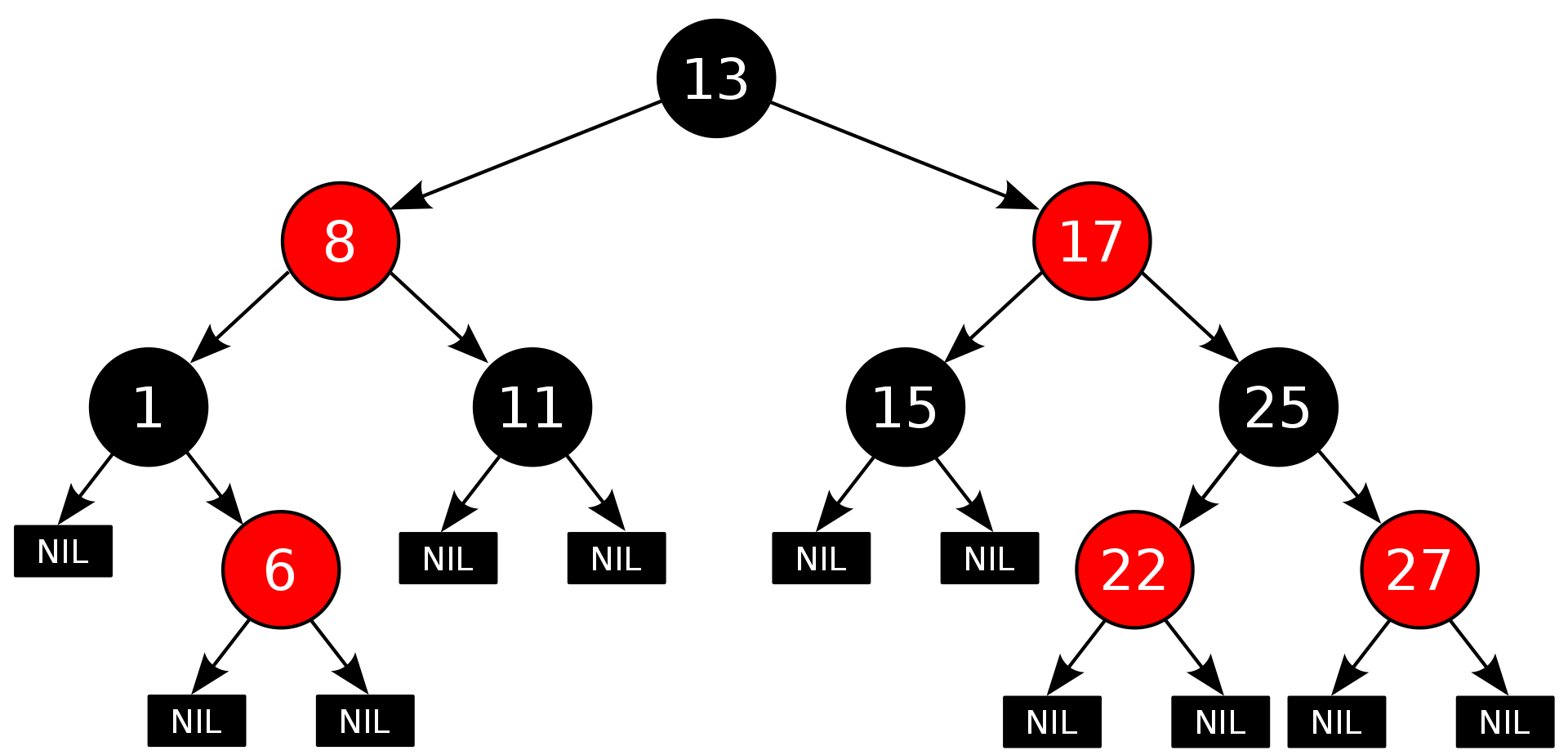这些约束确保了红黑树的关键特性：从根到叶子的最长的可能路径不多于最短的可能路径的两倍长。结果是这个树大致上是平衡的。 因为操作比如插入、删除和查找某个值的最坏情况时间都要求与树的高度成比例，这个在高度上的理论上限允许红黑树在最坏情况下都是高效的，而不同于普通的二叉查找树。
要知道为什么这些性质确保了这个结果，注意到性质4导致了路径不能有两个毗连的红色节点就足够了。最短的可能路径都是黑色节点，最长的可能路径有交替的红色和黑色节点。因为根据性质5所有最长的路径都有相同数目的黑色节点，这就表明了没有路径能多于任何其他路径的两倍长。
在树的结构发生改变时（插入或者删除操作），往往会破坏上述条件4或条件5，需要通过调整使得查找树重新满足红黑树的条件。
调整可以分为两类：一类是颜色调整，即改变某个节点的颜色；另一类是结构调整，集改变检索树的结构关系。结构调整过程包含两个基本操作：左旋（Rotate Left），右旋（Rotate Right）。
Java中TreeMap底层通过红黑树来实现，这样插入删除都只有$O(logn)$的时间复杂度。

### 4.1 (7)重建二叉树¶

Tip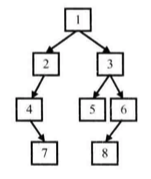private BinaryTreeNode construct(int[] preorder, int[] inorder, int length) throws Exception{
if (preorder == null || inorder == null || length <= 0) {
return null;
}

return constructCore(preorder, 0, preorder.length - 1, inorder, 0, inorder.length - 1);
}

private BinaryTreeNode constructCore(
int[] preorder, int startPreorder, int endPreorder,
int[] inorder, int startInorder, int endInorder
) throws Exception {
// 如果发生了这种情况，说明前中序子序列为空，那么肯定就是空节点了
if (startInorder > endInorder || startPreorder > endPreorder) {
return null;
}

// startPreorder即为根节点
BinaryTreeNode root = new BinaryTreeNode(preorder[startPreorder]);

boolean find = false;
int i = startInorder;
for (; i <= endInorder; i++) {
if (inorder[i] == root.value) {
find = true;
// 由于在中序序列中i是根节点，所以i-startInorder的长度就是左子树的长度
// 因此重建左子树时，左子树的前序序列范围就是startPreorder+1至startPreorder+1 + i-startInorder - 1 = startPreorder + i - startInorder
// 左子树的中序序列范围就是startInorder至i - 1，没有什么好说的
// 重建右子树时类似
root.left = constructCore(
preorder, startPreorder + 1, startPreorder + i - startInorder,
inorder, startInorder, i - 1);
root.right = constructCore(
preorder, startPreorder + i - startInorder + 1, endPreorder,
inorder, i + 1, endInorder);
}
}
// 最后，如果根节点在中序序列中没有找到，那么肯定是无效的输入了
if (!find) {
throw new Exception("invalid input");
}

return root;
}


### 4.2 (8)二叉树的下一个节点¶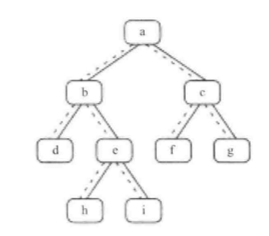• ，如果一个节点有右子树：那么它的下一个节点就是它的右子树中的最左子节点。也就是说，从右子节点出发一直沿着指向左子节点的指针，我们就能找到它的下一个节点。
• ，如果一个节点没有右子树，同时节点是它父节点的左子节点，那么它的父节点就是要找的下一个节点。
• ，如果一个节点没有右子树，同时节点是它父节点的右子节点：我们需要沿着父节点一直向上，直到找到一个是它父节点的左子节点的节点。这个父节点就是我们要找的下一个节点。
private ParentBinaryTreeNode getNext(ParentBinaryTreeNode node){
if (node == null) {
return null;
}

ParentBinaryTreeNode next = null;
if (node.right != null) {
// 情况1 从右子节点出发一直沿着指向左子节点的指针
next = node.right;

while (next.left != null) {
next = next.left;
}

return next;
} else if (node.parent != null) {
// 情况2、3可以理解为同一种 2是3的一般情况
next = node;

// 沿着父节点一直向上，直到找到一个节点，它是父节点的左子节点
// 即如果这个节点是父节点的右节点，那么继续寻找
while (next.parent != null && next == next.parent.right) {
next = next.parent;
}

// 最后这个父节点就是我们要找的下一个节点
return next.parent;
}

return next;
}


## 5. 栈和队列¶

• isEmpty
• peek
• pop
• push

• isEmpty
• peek
• poll
• offer

### 5.1 (9)用两个栈实现队列¶

class JQueue<T> {
private Stack<T> stack1 = new Stack<>();
private Stack<T> stack2 = new Stack<>();

public void appendTail(T element) {
stack1.push(element);
}

if (stack2.isEmpty()) {
while (!stack1.isEmpty()) {
stack2.push(stack1.pop());
}
}

if (stack2.isEmpty()) {
return null;
}

return stack2.pop();
}
}


/**
* 相关题目
* 用两个队列实现一个栈。
*/
class JStack<T> extends Stack<T>{
private Queue<T> queue1 = new LinkedList<>();
private Queue<T> queue2 = new LinkedList<>();

@Override
public T push(T item) {
queue1.offer(item);
return item;
}

@Override
public synchronized T pop() {
if (queue1.isEmpty()) {
throw new EmptyStackException();
}

while (queue1.size() > 1) {
queue2.offer(queue1.poll());
}

T item = queue1.poll();

Queue<T> tmp = queue1;
queue1 = queue2;
queue2 = tmp;

return item;
}

public static void main(String[] args) {
Stack<Integer> stack = new JStack<>();

stack.push(1);
System.out.println(stack.pop());
stack.push(2);
stack.push(3);
System.out.println(stack.pop());
stack.push(4);
System.out.println(stack.pop());
System.out.println(stack.pop());

// 输出 1 3 4 2
}
}


## 6. 算法和数据操作¶

### 6.1 递归和循环¶

#### 6.1.1 (10)斐波那契数列¶

private long Fibonacci_Solution1(int n) {
if (n == 0)
return 0;
else if (n == 1)
return 1;
else
return Fibonacci_Solution1(n - 1) + Fibonacci_Solution1(n - 2);
}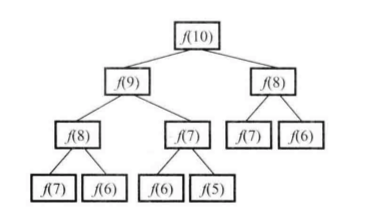private long Fibonacci_Solution2(int n) {
int[] results = {0, 1};
if (n < 2) {
return results[n];
}

int a = results;
int b = results;
int result = 0;

for (int i = 2; i <= n; i++) {
result = a + b;
a = b;
b = result;
}

return result;
}


\begin{bmatrix}f(n) & f(n-1) \\ f(n-1) & f(n-2)\end{bmatrix}=\begin{bmatrix}1 & 1 \\ 1 & 0\end{bmatrix}^{n-1}

a^n=\begin{cases} a^{n/2} \cdot a^{n/2}, & n为偶数 \\ a^{(n-1)/2} \cdot a^{(n-1)/2} \cdot a, & n为奇数 \end{cases}

private static class Matrix2By2 {
long m_00;
long m_01;
long m_10;
long m_11;

Matrix2By2(long m_00, long m_01, long m_10, long m_11) {
this.m_00 = m_00;
this.m_01 = m_01;
this.m_10 = m_10;
this.m_11 = m_11;
}

static Matrix2By2 matrixMultiply(Matrix2By2 matrix1, Matrix2By2 matrix2) {
return new Matrix2By2(
matrix1.m_00 * matrix2.m_00 + matrix1.m_01 * matrix2.m_10,
matrix1.m_00 * matrix2.m_01 + matrix1.m_01 * matrix2.m_11,
matrix1.m_10 * matrix2.m_00 + matrix1.m_11 * matrix2.m_10,
matrix1.m_10 * matrix2.m_01 + matrix1.m_11 * matrix2.m_11);
}

static Matrix2By2 MatrixPower(int n) {
Matrix2By2 matrix;

if (n == 1) {
matrix = new Matrix2By2(1, 1, 1, 0);
} else if (n % 2 == 0) {
matrix = MatrixPower(n / 2);
matrix = matrixMultiply(matrix, matrix);
} else { // n % 2 == 1
matrix = MatrixPower((n - 1) / 2);
matrix = matrixMultiply(matrix, matrix);
matrix = matrixMultiply(matrix, new Matrix2By2(1, 1, 1, 0));
}

return matrix;
}
}

private long Fibonacci_Solution3(int n) {
int[] results = {0, 1};
if (n < 2) {
return results[n];
}

Matrix2By2 matrix2By2 = Matrix2By2.MatrixPower(n - 1);
return matrix2By2.m_00;
}


$f(8)=f(7)+f(6)$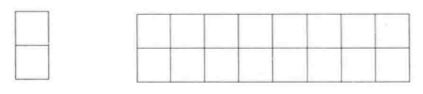### 6.2 查找和排序¶

public class QuickSort {

private static int partition(int[] numbers, int lo, int hi) {
if (numbers == null || numbers.length == 0 || lo < 0 || hi >= numbers.length) {
throw new IllegalArgumentException("Invalid params");
}

int key = numbers[lo];

while (lo < hi) {
// 比选择的数字小的数字移到数组左边
while (numbers[hi] >= key && hi > lo) {
hi--;
}
numbers[lo] = numbers[hi];

// 比选择的数字大的移到数组右边
while (numbers[lo] <= key && hi > lo) {
lo++;
}
numbers[hi] = numbers[lo];
}
numbers[hi] = key;

return hi;
}

public static void sort(int[] numbers, int lo, int hi) {
if (lo >= hi) {
return;
}
int index = partition(numbers, lo, hi);
sort(numbers, lo, index - 1);
sort(numbers, index + 1, hi);
}

public static void main(String[] args) {
int[] numbers = {4, 3, 1, 5, 6, 10, 7, 6, 8};
sort(numbers, 0, numbers.length - 1);

for (int num : numbers) {
System.out.print(" " + num);
}
}
}


public static void sort(Comparable[] a) {
// 将a[]升序排列
int N = a.length;
for (int i = 0; i < N; i++) {
for (int j = i; j > 0 && AbsSort.less(a[j], a[j-1]); j--) {
AbsSort.exch(a, j, j-1);
}
}
}


public static void sort(Comparable[] a){
// 将a[]升序排列
int N = a.length;
for (int i = 0; i < N - 1; i++) {
for (int j = 0; j < N - i - 1; j++) {
if (AbsSort.less(a[j], a[j+1])) {
AbsSort.exch(a, j, j+1);
}
}
}
}


public class Merge extends AbsSort{
private static Comparable[] aux;        // 归并所需的辅助数组

public static void sort(Comparable[] a) {
aux = new Comparable[a.length];
sort(a, 0, a.length - 1);
}

private static void sort(Comparable[] a, int lo, int hi) {
if (hi <= lo) return;
int mid = lo + (hi - lo)/2;
sort(a, lo, mid);
sort(a, mid+1, hi);
merge(a, lo, mid, hi);
}

/**
* 原地归并
*/
public static void merge(Comparable[] a, int lo, int mid, int hi) {
int i = lo, j = mid+1;

for (int k = lo; k <= hi; k++)
aux[k] = a[k];

for (int k = lo; k <= hi; k++) {
if (i > mid)                        a[k] = aux[j++];
else if (j > hi)                    a[k] = aux[i++];
else if (less(aux[j], aux[i]))      a[k] = aux[j++];
else                                a[k] = aux[i++];
}
}
}


public class MergeBU extends AbsSort{
private static  Comparable[] aux;

public static void sort(Comparable[] a) {
int N = a.length;
aux = new Comparable[N];
for (int sz = 1; sz < N; sz = sz+sz)                    // sz子数组大小
for (int lo = 0; lo < N-sz; lo += sz+sz)            // lo:子数组索引
merge(a, lo, lo+sz-1, Math.min(lo+sz+sz-1, N-1));
}

/**
* 原地归并
*/
public static void merge(Comparable[] a, int lo, int mid, int hi) {
int i = lo, j = mid+1;

for (int k = lo; k <= hi; k++)
aux[k] = a[k];

for (int k = lo; k <= hi; k++) {
if (i > mid)                        a[k] = aux[j++];
else if (j > hi)                    a[k] = aux[i++];
else if (less(aux[j], aux[i]))      a[k] = aux[j++];
else                                a[k] = aux[i++];
}
}
}


Sorting algorithm

#### 6.2.1 (11)旋转数组的最小数字¶

int min(int[] numbers) {
if (numbers == null) {
throw new IllegalArgumentException("Invalid params");
} else if (numbers.length == 1) {
return numbers;
}

int lo = 0;
int hi = numbers.length - 1;

while (lo < hi) {
int mid = (lo + hi) >> 1;

// 当lo，mid，hi对应的数字都相等时，采用顺序查找
if (numbers[lo] == numbers[mid] &&
numbers[mid] == numbers[hi]) {
return minInOrder(numbers, lo, hi);
}

if (numbers[mid] > numbers[hi]) {
// a[mid]>a[hi]，说明最小值在右边，且肯定不会是a[mid]，因为a[mid]>a[hi]>=min
lo = mid + 1;
} else {
// a[mid]<=a[hi]，最小值可能是mid，所以不能漏掉
hi = mid;
}
}

return numbers[hi];
}

private int minInOrder(int[] numbers, int start, int end) {

int min = numbers[start];
for (int i = start + 1; i <= end; i++) {
if (numbers[i] < min) {
min = numbers[i];
return min;
}
}

return min;
}


### 6.3 回溯法¶

#### 6.3.1 (12)矩阵中的路径¶

A \underline{B} T G \\ C \underline{F} \underline{C} S \\ J D \underline{E} H

private int pathLength = 0;

private boolean hasPath(String matrix, int rows, int cols, String str) {
if (matrix == null || matrix.length() != rows * cols || str == null) {
return false;
}
boolean[] visited = new boolean[matrix.length()];

pathLength = 0;

for (int row = 0; row < rows; row++) {
for (int col = 0; col < cols; col++) {
if (hasPathInner(matrix, rows, cols, row, col, str, visited)) {
return true;
}
}
}

return false;
}

private boolean hasPathInner(
String matrix, int rows, int cols, int row, int col, String str, boolean[] visited
) {
if (pathLength == str.length()) {
return true;
}

boolean hasPath = false;
if (row >= 0 && row < rows && col >= 0 && col < cols
&& matrix.charAt(row * cols + col) == str.charAt(pathLength)
&& !visited[row * cols + col]) {
pathLength++;

visited[row * cols + col] = true;

hasPath = hasPathInner(matrix, rows, cols, row, col - 1,
str, visited)
|| hasPathInner(matrix, rows, cols, row - 1, col,
str, visited)
|| hasPathInner(matrix, rows, cols, row, col + 1,
str, visited)
|| hasPathInner(matrix, rows, cols, row + 1, col,
str, visited);

if (!hasPath) {
pathLength--;
visited[row * cols + col] = false;
}
}

return hasPath;
}


#### 6.3.2 (13)机器人的运动范围¶

private int movingCount(int threshold, int rows, int cols) {
if (threshold < 0 || rows <= 0 || cols <= 0) {
return 0;
}

boolean[] visited = new boolean[rows * cols];

return movingCountInner(threshold, rows, cols, 0, 0, visited);
}

private int movingCountInner(int threshold, int rows, int cols, int x, int y, boolean[] visited) {
int index = rows * x + y;

int count = 0;
if (x >= 0 && x < cols && y >= 0 && y < rows && !visited[index] && isMatched(threshold, x, y)) {
visited[index] = true;
count =
movingCountInner(threshold, rows, cols, x - 1, y, visited)
+ movingCountInner(threshold, rows, cols, x, y - 1, visited)
+ movingCountInner(threshold, rows, cols, x + 1, y, visited)
+ movingCountInner(threshold, rows, cols, x, y + 1, visited)
+ 1;
}
return count;
}

private boolean isMatched(int threshold, int x, int y) {
int sum = 0;
do {
sum += x % 10;
x /= 10;
} while (x != 0);
do {
sum += y % 10;
y /= 10;
} while (y != 0);

return sum <= threshold;
}


### 6.4 动态规划与贪婪算法¶

#### 6.4.1 (14)剪绳子¶

private int maxProductAfterCutting_solution1(int length) {
if (length < 2) {
return 0;
} else if (length == 2) {
return 1;
} else if (length == 3) {
return 2;
}

int[] products = new int[length + 1];
products = 0;
products = 1;
products = 2;
products = 3;

for (int i = 4; i <= length; i++) {
int max = 0;
for (int j = 1; j <= i / 2; j++) {
int result = products[j] * products[i - j];
if (result > max) {
max = result;
}
products[i] = max;
}
}

return products[length];
}


private int maxProductAfterCutting_solution2(int length) {
if (length < 2) {
return 0;
} else if (length == 2) {
return 1;
} else if (length == 3) {
return 2;
}

// 尽可能多剪去长度为3的绳子段
int timesOf3 = length / 3;

// 当绳子最后剩下的长度为4时，不能再减去长度为3的绳子段
if (length - timesOf3 * 3 == 1) {
timesOf3--;
}
// 此时更好的方法是把绳子剪成长度为2的两段，因为2x2>3x1
int timesOf2 = (length - timesOf3 * 3) / 2;

return (int) Math.pow(3, timesOf3) * (int) Math.pow(2, timesOf2);
}


### 6.5 位运算¶

00001010 << 2 = 00101000
10001010 << 3 = 01010000

00001010 >> 2 = 00000010
10001010 >> 3 = 11110001

### 6.5.1 (15)二进制中1的个数¶

int NumberOf1_Solution1(int n) {
int count = 0;
int flag = 1;

while (flag != 0) {
if ((n & flag) != 0) {
count++;
}

flag = flag << 1;
}

return count;
}


1100 -> (1011 & 1100) = 1000 -> 0
1010 -> (1001 & 1010) = 1000 -> 0

int NumberOf1_Solution2(int n) {
int count = 0;

while (n != 0) {
count++;
n = (n - 1) & n;
}

return count;
}